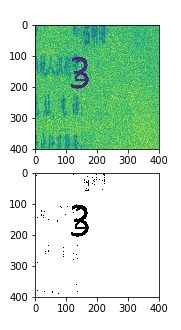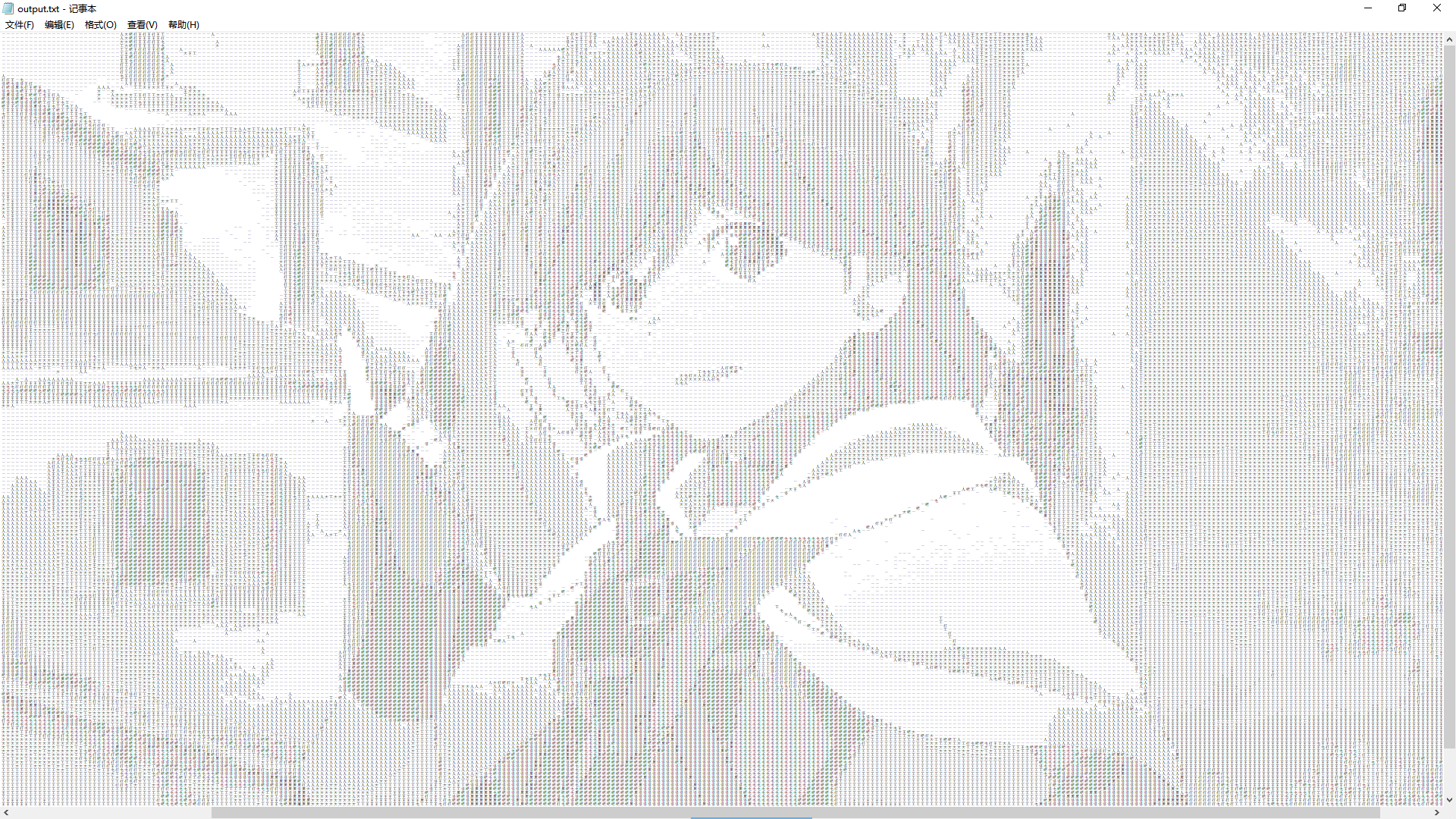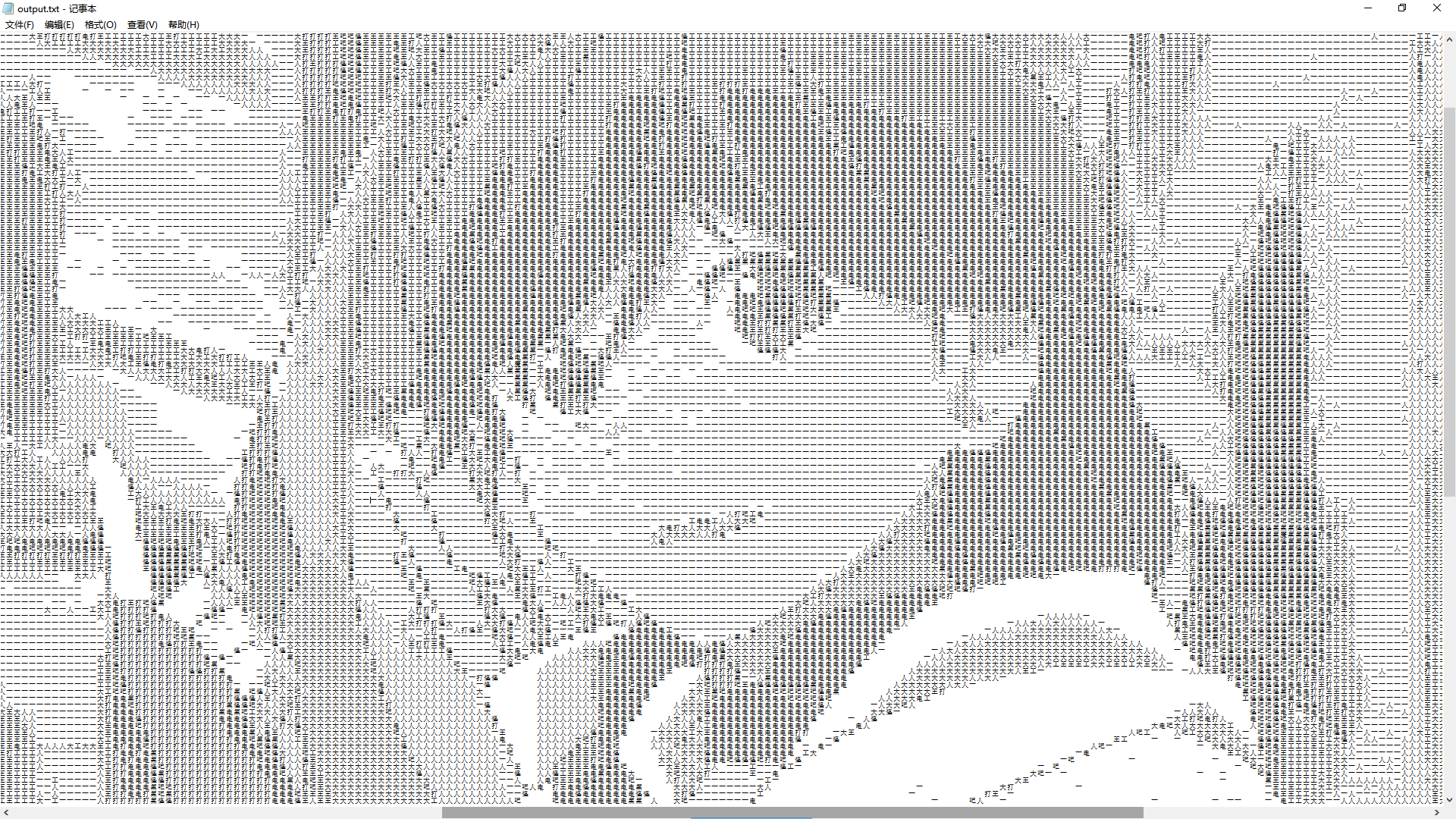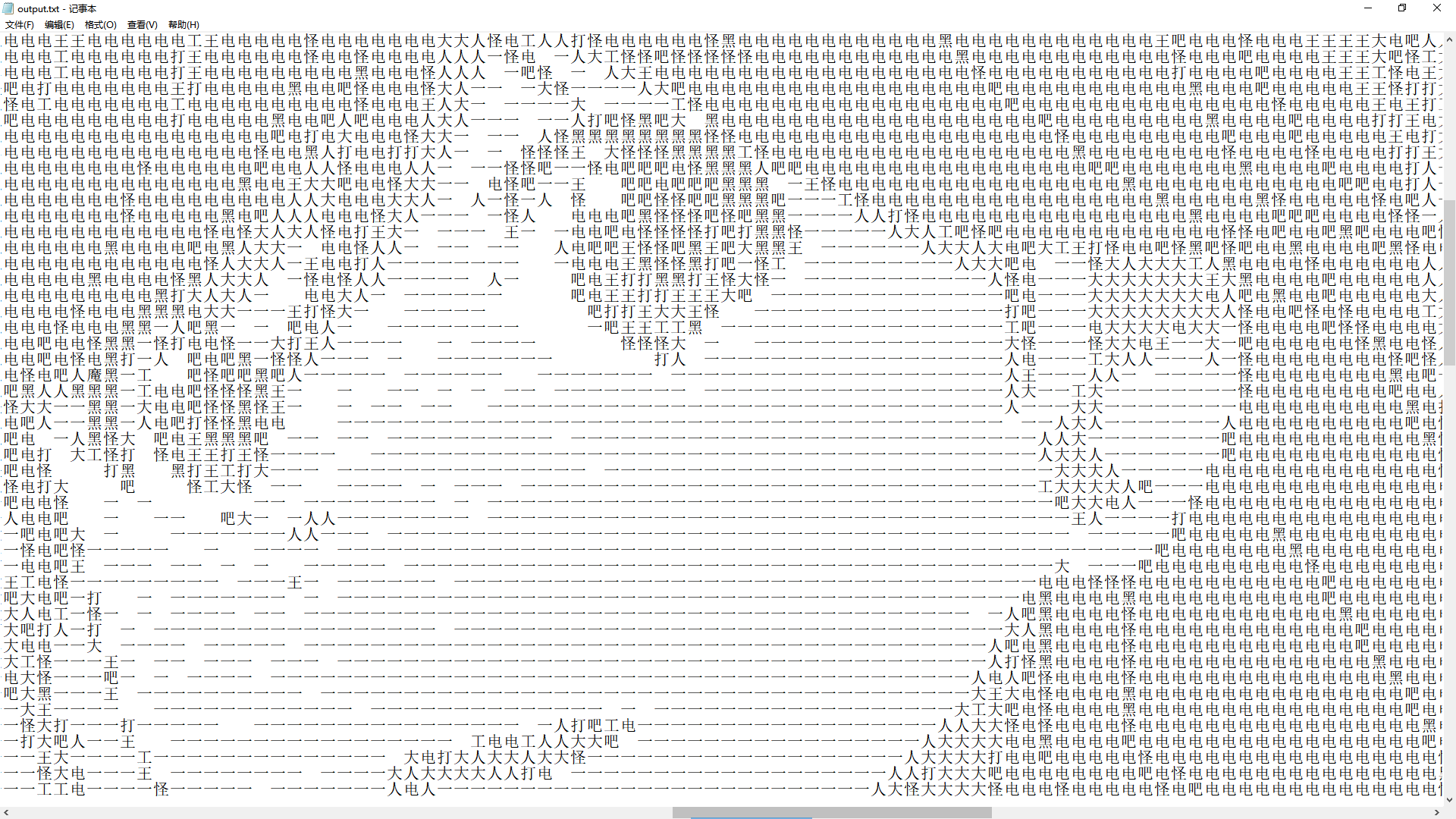• 图像可以分为RGB（3通道，每个通道 的值是0-255），灰度图（单通道，值范围0-255），二值图（0：黑色，1：白色）。 fig,ax = plt.subplots(2,1,figsize = (5,5)) ax.imshow(tmp) ax.imshow(tmp2,cmap = ...
1 理论
图像可以分为RGB（3通道，每个通道 的值是0-255），灰度图（单通道，值范围0-255），二值图（0：黑色，1：白色）。
fig,ax = plt.subplots(2,1,figsize = (5,5))
ax.imshow(rgbFig)
ax.imshow(rgbFig,cmap = "gray")

加了“gray”，就显示二值。RGB转换为灰度图的方法：
import os
# 更换工作目录
os.chdir(r'F:\result')

from PIL import Image
# 读取RGB图片并转换为灰度图
img = Image.open('myrgbimg.png').convert('LA')
plt.imshow(img)
plt.show()
img.save('greyscale.png')

其他： 彩色照片转黑白图片 在线网址

参考：
How can I convert an RGB image into grayscale in Python?;
展开全文• from skimage import io,transform,color import numpy as np def convert_gray(f,**args):#图片处理与格式化的函数 ... gray=color.rgb2gray(rgb)#将彩色图片转换为灰度图片 dst=transform.resize(gr...
from skimage import io,transform,color
import numpy as np

def convert_gray(f,**args):#图片处理与格式化的函数
gray=color.rgb2gray(rgb)#将彩色图片转换为灰度图片
dst=transform.resize(gray,(64,64))#调整大小，图像分辨率为64*64
return dst
datapath='D:/input_image3'#图片所在的路径
str=datapath+'/*.jpg'#识别.jpg的图像
for i in range(len(coll)):
io.imsave(r'd:/input_image4/'+np.str(i)+'.bmp',coll[i]) #保存图片在d:/input_image4

展开全文opencv 人脸识别 图像识别 计算机视觉
• 本文实例为大家分享了python实现彩色图转换成灰度图的具体代码，供大家参考，具体内容如下 from PIL import Image import os # 图像组成：红绿蓝 （RGB）三原色组成 亮度（255,255,255） image = "Annie1.jpg" img ...
• ...5.关闭程序，打开放图片的目录，发现会多出来一个output.txt文档，打开，字体调小即可看到文字   原（1920*1080）： 效果（384*216）： 放大： 再放大：

from PIL import Image
import argparse
parser = argparse.ArgumentParser()
args = parser.parse_args()
IMG = args.file
WIDTH = args.width
HEIGHT = args.height
OUTPUT = args.output
ascii_char = list("魔吧打王大工人一")    # 可自定义字符种类，但注意灰度要递减

def get_char(r, g, b, alpha = 256):
if alpha == 0:
return ' '
length = len(ascii_char)
gray = int(0.2126 * r + 0.7152 * g + 0.0722 * b)    # 灰度参数，这个适用于二次元图片，其他请自行调参

unit = (256.0 + 1)/length
return ascii_char[int(gray/unit)]

if __name__ == '__main__':
im = Image.open(IMG)
im = im.resize((WIDTH,HEIGHT), Image.NEAREST)
txt = ""
for i in range(HEIGHT):
for j in range(WIDTH):
txt += get_char(*im.getpixel((j,i)))
txt += '\n'
print(txt)
if OUTPUT:
with open(OUTPUT, 'w') as f:
f.write(txt)
else:
with open("output.txt", 'w') as f:
f.write(txt)

操作步骤：
1.下载pycharm和sdk，按照百度教程配置环境变量。
2.下载pip包，pillow包。
3.把该代码的py文件和要转化的图片放在电脑C盘用户名目录下
4.打开cmd窗口，输入命令   python 1.py 1.png    （后两项分别是py文件名和图片名)
5.关闭程序，打开放图片的目录，发现会多出来一个output.txt文档，打开，把字体调小即可看到文字图

原图（1920*1080）：效果图（384*216）：放大：再放大：展开全文转化 代码
• 今天小编就为大家分享一篇python-OpenCV 实现将数组转换成灰度图和彩图，具有很好的参考价值，希望对大家有所帮助。一起跟随小编过来看看吧
• #coding=utf-8 from PIL import Image I = Image.open('JINSHA.jpg') I.show() L = I.convert('L') L.show() ps：最重要的是注意文件路径
#coding=utf-8
from PIL import Image
I = Image.open('JINSHA.jpg')
I.show()
L = I.convert('L')
L.show()
ps：最重要的是注意文件路径
展开全文• from PIL import Image import os file_dir = '替换为输入路径' out_dir = '替换为输出路径' a = os.listdir(file_dir) for i in a: print(i) I = Image.open(file_dir+i) ... L.save(out_di...
• python 灰度图片转二值图片 img = cv2.imread("G:/imgHui/jiaoshi.jpg") Grayimg = cv2.cvtColor(img, cv2.COLOR_BGR2GRAY) ret, thresh = cv2.threshold(Grayimg, 120, 225,cv2.THRESH_BINARY) cv2....
• 注意这里可以写绝对路径 也可以像本代码一样写相对路径，在写相对路径时应该将图片和代码置于同一个文档目录底下。以下是代码： ...CR7_gray就是转换后的灰度图，也保存在域代码相同的文档目录下 ...
• 模式 1 1位像素，黑和白，存8位的像素 L 8位像素，黑白 P 8位像素，使用调色板映射到任何其他模式 RGB 3×8位像素，真彩 RGBA 4×8位像素，真彩+透明通道 CMYK 4×8位像素，颜色隔离 YCbCr 3×8位像素，彩色视频...
• 在实现彩色图片转换为灰度图片之前，先来了解一下图像数字化。当你张开眼睛，就看到现实的世界，比如房子，花草和不同的物品。如果要当前的眼睛所见的东西保存起来，在以前是使用胶片相机，那是采用化学的感光方法...opencv
• 在进行深度学习之前，往往需要创建一个用于训练的图片数据集，且一般要求训练集具有统一 的格式和大小。本文以批量处理大小不一 的jpg图片为例，介绍批量处理的一些问题。方法仅供参考。 import os ...深度学习 批量处理
• ## python opencv将图片转为灰度图

万次阅读 多人点赞 2018-12-05 23:00:23
使用opencv将图片转为灰度图主要有两种方法，第一种是将彩色图转为灰度图，第二种是在使用OpenCV读取图片的时候直接读取为灰度图。 将彩色图转为灰度图 import cv2 import numpy as np if __name__ == "__main...
• 然而从网上下载的图片大多是彩色图，所以就需要彩色图转换成灰度图，同时要实现批处理。我选择用python语言来编程实现批量将彩色图转为灰度图。代码如下，有注释。from skimage import io,transform,colorimport ...
• 1.生成普通python数组(bytearray(),os.urandom()) 2.转换numpy数组(numpy.array()) 3.通过reshape将数组转换到所需的维数 4.以图像的形式显示出来(cv.imshow()) 代码 import os import cv2 as cv import numpy...OpenCV
• 使用opencv将图片转为灰度图主要有两种方法，第一种是将彩色图转为灰度图，第二种是在使用OpenCV读取图片的时候直接读取为灰度图。 将彩色图转为灰度图 import cv2 import numpy as np if __name__ == __main__: ...
• import cv2 img = cv2.imread("***....gray = cv2.cvtColor(img, cv2.COLOR_BGR2GRAY) # 转化为灰度图 cv2.imshow("src", gray) # 输出 dst = cv2.equalizeHist(gray) # 灰度图均衡化 cv2.imshow("gray", dst)...图像处理
• 下面小编就为大家带来一篇Python 将RGB图像转换为Pytho灰度图像的实例。具有很好的参考价值。希望对大家有所帮助。一起跟随小编过来看看吧
• 看网上有很多python环境下对rgb灰度化的处理方法，其中我感觉比较简单的一种方式是使用PIL进行灰度化处理： from PIL import Image I = Image.open(’***.jpg’) I.show() L = I.convert(‘L’) L.show() 或者 from ...PIL Pillow 机器学习
• 下面代码实现了input文件夹下所有彩色图像转换成灰度图像，如果要转换黑白图像只需L = I.convert('1')换L = I.convert('1') from PIL import Image import os input_dir = 'input/' out_dir = 'output/'...图像处理
• 1.将RGB图像转换为灰度图像： from PIL import Image I = Image.open('F:\\pycharm\\picture_format\\data\\lena.jpg') I.show() L = I.convert('L') L.show() 输出图像结果为： 2.将RGB图像转换为1...图像格式转换
• 本文主要是讲OpenCV-Python对图像进行彩色转灰度图的操作 文章目录前言一、读取图片二、彩色图片转灰度图强烈说明： 前言 这里给出一个OpenCV-Python官方文档的链接 链接: OpenCV-Python官方文档. 一、读取图片 ...opencvImage numpy 图像处理
• 想不到，今天学习的竟然还是彩色图像...经过前面的学习，你们和我一样，有没有掌握 2 种彩色图像转换成灰度图像的方式，2021年第一天在学习1种。 取经之旅第 8 天彩色图像转换为灰度图像伪彩色图像感谢大佬方向性的指导opencv 计算机视觉 人工智能 图像处理
• ref:https://www.cnblogs.com/Chilly2015/p/4943724.html ... RGB到灰度图转换公式： Y' = 0.299 R + 0.587 G + 0.114 B import numpy as np import ...
• python 批量将图片转为灰度图 from PIL import Image import os path = 'F:/QUEXIANJIANCESHIYAN/posdata' file_list = os.listdir(path) for file in file_list: I = Image.open(path+"/"+file) L = I.convert('L...opencv...

# 把图片转成灰度图pythonpython 订阅